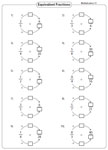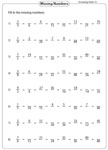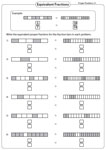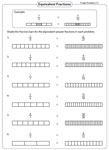1. Worksheets>
2. Math>
3. Pre-Algebra>
4. Fractions>
5. Equivalent Fractions

# Equivalent Fractions Worksheets

Equivalent fraction worksheets contain step-by-step solving process, identifying missing numbers, finding the value of the variables, completing the chain of equivalent fractions, writing equivalent fractions represented by pie models and fraction bars and representing the visual graphics in fractions. Explore some of these worksheets for free.

Step-by-Step Solving

Each printable worksheet has ten problems finding equivalent fractions by undergoing a step-by-step process.Multiplication:

Division:

Mixed:

Missing Number: Standard

Find the missing number that makes the equivalent fractions. Easy level has 2, 3, 4 and 5 as factors. Moderate level has factors between 1 and 11. Difficult level contains factors in the range 2-25.Easy:

Moderate:

Difficult:

Missing Number: Variables

Find the value of the variables that makes the equivalent fractions. You may use cross-multiplication method to find the values.Easy:

Moderate:

Difficult:

Each question has 8 equivalent fractions. Using the first fraction, complete the chain of equivalent fractions.Increasing:

Decreasing:

Mixed:

Equivalent Fraction Pattern

Each question has a bunch of equivalent fractions. Find the missing equivalent fraction by identifying the pattern followed by both the numerators and denominators.Easy (5 terms):

Moderate (8 terms):

Who am I?

Hey! I give you the clue. Can you find what fraction am I? Type-2 have fractions expressed in word forms.Type 1:

Type 2:

Mixed:

Identifying Equivalent Fractions

Each pdf worksheet has two sections. First section contains six multiple choices. Second section contains questions on writing your own equivalent fractions.Equivalent or Not Equivalent

Insert the correct symbol to show the pair of pizza fractions are equivalent or not equivalent.Visuals:

Standard:

Writing Equivalent fractions: Pie Model

Each question in this batch of pdf worksheets has a pair of equivalent pie models. Express the pizza models in equivalent fractions.Proper Fractions:

Improper Fractions:

Mixed Numbers:

Writing Equivalent fractions: Bar Model

Look at the equivalent fraction bars and write the fractions represented by them.Proper Fractions:

Improper Fractions:

Mixed Numbers:

Representing Equivalent fractions: Pie Model

Shade the pie wedges to represent the equivalent fractions in these printable pdfs. Ask the children to color the visual pie graphics (not compulsory).Proper Fractions:

Improper Fractions:

Mixed Numbers:

Representing Equivalent fractions: Bar Model

Color (or shade) the fraction bar to represent each fraction. See how they are equivalent.Proper Fractions:

Improper Fractions:

Mixed Numbers:

Related Worksheets